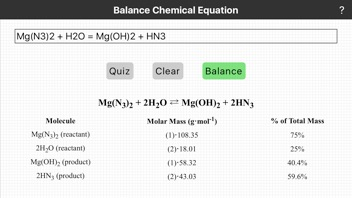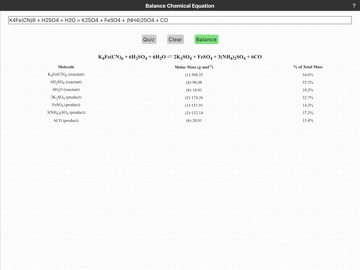### iPhone Screenshots### Description

In a reaction chemical bonds are broken and formed. In the process, atoms are rearranged into new molecules, but are neither created nor destroyed. A chemical reaction can be represented as an equation: reactants = products. By substituting the chemical formulas for the reactants and products we obtain a qualitative summary of a reaction.
A balanced, quantitative, chemical equation has the same numbers of atoms of each element on the two sides of the equation ( law of conservation of matter ). To balance an equation we add stoichiometric coefficients in front of constituent molecules.
The word stoichiometric is derived from the Greek words for "element" and "measure." Stoichiometry defines the quantitative relationship between the relative quantities (numbers of moles) of reactants and products taking part in a chemical reaction.
In some cases, balancing a chemical equation by hand is easy. In general as the number of elements and constituent molecules increases so does the difficulty of balancing the equation.
To use this App enter an equation and tap Balance. Separate reactants and products with =. The Quiz feature lets you practice balancing equations by hand. Tap Quiz to display an unbalanced chemical equation. Work out your answer, then check the result.

### What's New in Version 1.2.2

+50 new practice equations. Updated code base.

### Seller

#### Name

Thundercloud Consulting, LLC

1.2.2

4.4 MB

No

Yes

Rated: 4+

iOS 8.0 or later

English

No rating

No Entry